français | español | polski | 日本語 |

Pokemon Math Quiz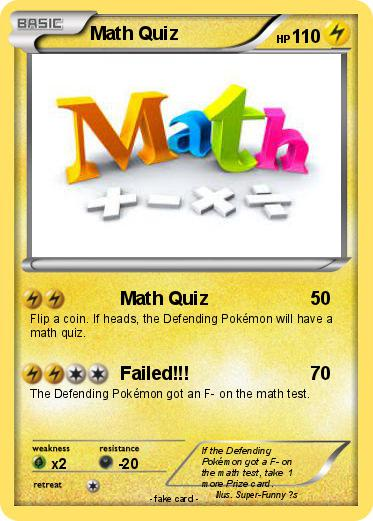Language Card : english

Creation Date : 18 February 2016

### Pokemon Passport

Name : Math Quiz

Serie : Black & White

Type : Lighting

Attack 1 : Math Quiz
Flip a coin. If heads, the Defending Pokémon will have a math quiz.

Attack 2 : Failed!!!
The Defending Pokémon got an F- on the math test.

Comments : If the Defending Pokémon got a F- on the math test, take 1 more Prize card.

Illustrator : Super-Funny ?s

### Vote for this card

(6 votes)

Related cards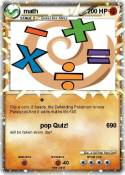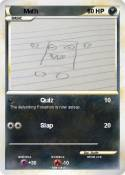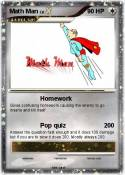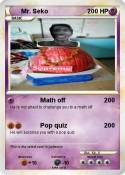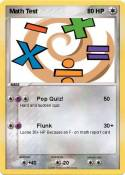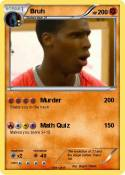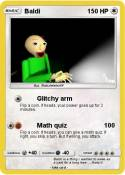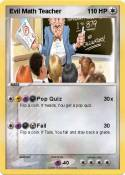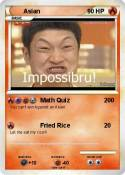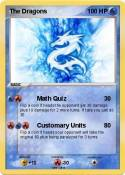Dzvinka | Mr. Busse | The Impossible Quiz | Vote 5 if you like math | Vote if u H8 MATH bad as diz face | Math Lover | math 111111111111111112 | Math teacher | math mega | Sinapore Math 3B | JiJi ST math | The Great Math Wolf | Foxeh the math Fox | Khan academy | Vote 5 if you hate math | Math Mann | I Can Do Math Everyday! | MATH | Math Teacher | MATh | math team | Math Book | Math knight | Brice | Math | Math Teacher | Math :( | math 100 x | me in math | Math man | Daniel EX | Nerd | math | Math Homework | Cool Math | math doesn't lie | Tushar | Math 24 | Math Knight | Math Man | Math | Boring Math Teacher | Math | Mrs Ferriola | 1+1 | Ratey hte math cat | St Math | Mrs Baker | baldi | Math | Math | Math | Math | more math | math | Asian guy | Asian guy | School | Math | ?????? | Quiz | Mettaton | mystery | Quizilla | Blaine | school | Scout | gina | Teacher | Impossible Quiz

Comments

Pokémon is a registered trademark of Nintendo, Creatures, Game Freak and The Pokémon Company
MyPoKeCaRd.cOm 0.9 - Propulsed by jquery, php, gd and spip - XHTML 1 and CSS2 valid page
MyPokeCard.com is a funny site to design your own pokemon card, vote for the best pokemon cards and create pokemon colorings